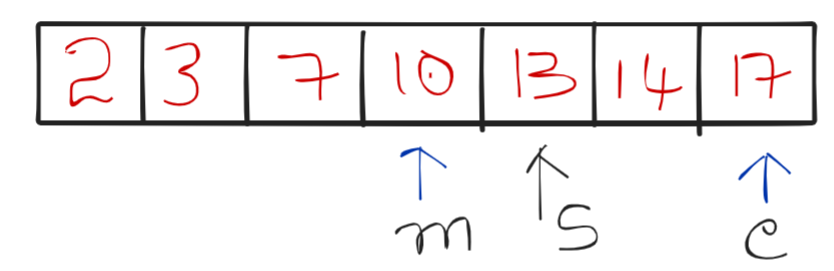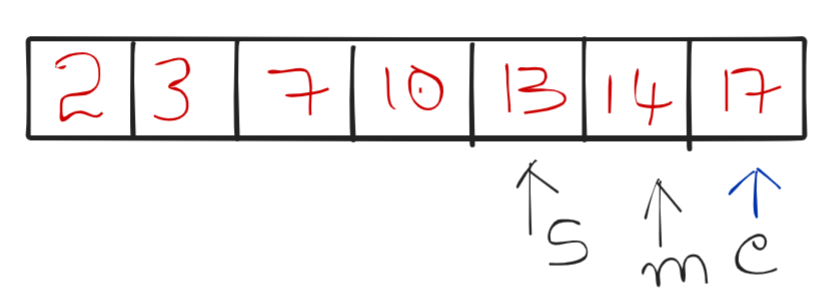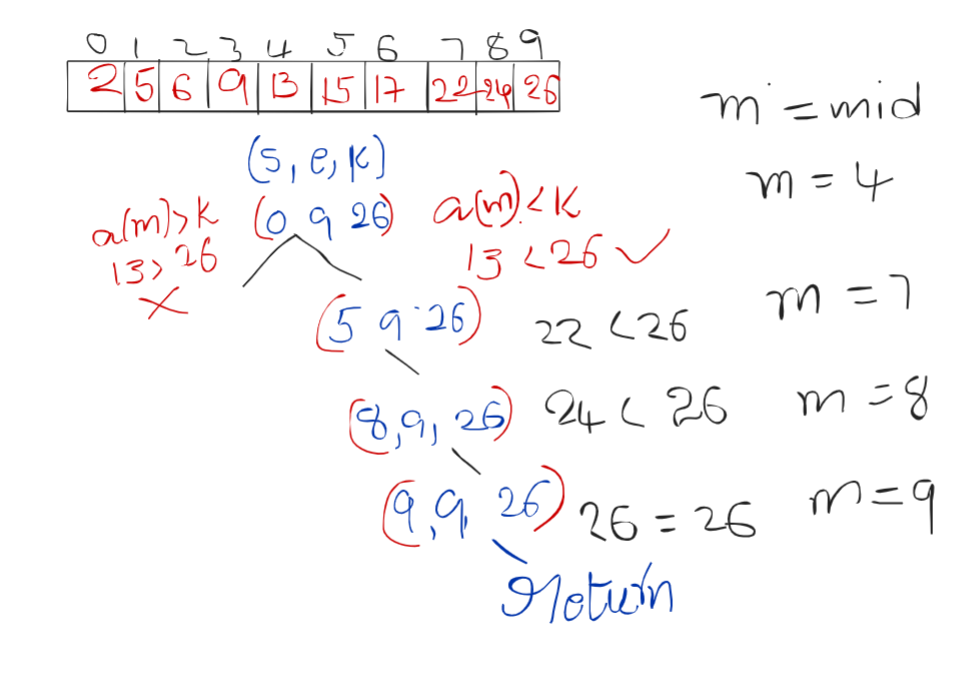# Binary Search : Explained

In this article we will learn about one of most easy yet important searching algorithm “Binary Search”.

Let’s consider this simple problem statement: You are given an array of integers and a key element, your task is to search for the key in the given array.

One simple approach you can follow is to traverse through the entire array and while traversing compare every element with the key, if the key is a match with current array element then you are done with your task.

But what if the given array length is very large? If you check every element with a key then it will take more time which is not optimal.

So, to optimise the search operation we will use “Binary Search”, that is an algorithm for searching and uses the “Divide and Conquer” technique.

Note: Binary Search will work only on Sorted numbers

Divide and Conquer:

• Break down the problem into subproblems
• Solve the sub problems
• Merge the sub problems to get desired Output

Intuition and Thought process for Binary search:

Let’s say you are given 100 balls which are numbered from 1 to 100 and they are in increasing order and your task is to pick a ball which has 80 numbered on it, now where will you look for this 80th ball? Will you start looking for this ball from the 1st ball and check every ball whether the ball you picked is the 80th ball or not OR will you directly look for the last part of the balls? You will definitely look at the last part of the balls because you know balls are in increasing order. So you may look after the middle part of the entire ball which maybe after 50 or 60 balls to search for the 80th ball.

In the same way, a Binary search algorithm will work, This algorithm will look for the number by dividing the array into half and check if the middle element is greater/lesser/equal to the Key value if the key is less than the middle element then the algorithm will look for key only from starting element to middle-1 position element, else if the key is greater than the mid then it will look from middle element +1 index position element to last element, else if the key is equal to the middle then the algorithm will terminate their itself. This process continues for every half of the array till starting index is less than the ending index.

This is how binary search works.

Algorithm:

• Consider start index to be at 0 and last index to be n-1th index at starting      //n->length
• Find middle index(mid) of the array
• If key is found to be less than mid index element then update last index of the array to mid -1
• Else if key is found to be greater than mid index element then update start index of the array to mid +1
• Else check for mid index element with key if not match repeat the above steps till start index is less than end index

Let’s see pictorially how it works:

Say we have array[] = {2,3,7,10,13,14,17}

Key =14;

Key is 14 > array[mid] which is (10)
So make start = mid+1;Mid = (4+6)/2 = 10/2 = 5Now the key is not > array[mid] and not < array[mid] but it is equal to array[mid], So algorithm stops here.

Iterative code:

## C++ Code

``````#include<bits/stdc++.h>
using namespace std;

int main() {
int arr[]= {2,3,7,10,13,14,17};
int k = 14;

//Binary search
int n = 6;
int start =0, end=n;
int mid,loc=-1;
while (start<=n-1){
//Making array half everytime
mid=(start+end)/2;

//checking in which part the element is present
if (arr[mid]<k){
start=mid+1;
}
else if (arr[mid]>k){
end=mid-1;
}
if (arr[mid]==k)
{
loc = mid;
break;
}
}
if (loc==-1){
}
else {
cout<<"Element " << k << " Found at " << loc << " index";
}

}

``````

Output: Element 14 Found at 5 index

Time complexity: O(log n)

Space complexity : O(1)

## Java Code

``````public class BinarySearch {

public static void main(String[] args) {
int arr[]= {2,3,7,10,13,14,17};
int k = 14;

//Binary search
int n = arr.length-1;
int start =0, end=n;
int mid,loc=-1;
while (start<=n-1){
//Making array half everytime
mid=(start+end)/2;

//checking in which part the element is present
if (arr[mid]<k){
start=mid+1;
}
else if (arr[mid]>k){
end=mid-1;
}
if (arr[mid]==k)
{
loc = mid;
break;
}
}
if (loc==-1){
}
else {
System.out.println("Element " + k + " Found at " + loc + " index");
}

}
}
``````

Output: Element 14 Found at 5 index

Time complexity: O(log n)

Space complexity : O(1)

Recursive Approach:

Intuition and approach: In the iterative code we are making the array half using a while loop every time, in the same way, we can half the array by passing the indices to the function again and again.

While halving the array by calling functions again and again we will check for conditions that we checked in iterative code.

Algorithm

• If start is less than end perform Binary search else terminate the algorithm.
• If the element at the middle index is equal to the key then return the index as it found the key
• Else if the key is less than the element at the middle index then call the function by passing end as mid-1 (as the key will be less than mid element)
• Else if the key is greater than the element at the middle index then call the function by passing start as mid+1 (as the key will be greater than mid)

Recursive tree: Key – 26Recursive Code:

## C++ Code

``````#include<bits/stdc++.h>

using namespace std;

int binarySearch(int arr[], int start, int end, int k) {

if (start > end) {
return -1;
}
int mid = (start + end) / 2;

if (k == arr[mid]) {
return mid;
} else if (k < arr[mid]) {
return binarySearch(arr, start, mid - 1, k);
} else {
return binarySearch(arr, mid + 1, end, k);
}
}

int main() {
int arr[] = {2,3,7,10,13,14,17};

int k = 14;

int start = 0;
int end = 6;

int loc = binarySearch(arr, start, end, k);

if (loc == -1) {
cout << "Element not found!" << endl;
} else {
cout << "Element " << k << " Found at " << loc << " index";
}

}``````

Output:

Element 14 Found at 5 index

Time complexity: O(log n)

Space complexity: O(logn) for auxiliary space

## Java Code

``````public class BinarySearch {

public static int binarySearch(int[] arr, int start, int end, int k) {

if (start > end) {
return -1;
}
int mid = (start + end) / 2;

if (k == arr[mid]) {
return mid;
} else if (k < arr[mid]) {
return binarySearch(arr, start, mid - 1, k);
} else {
return binarySearch(arr, mid + 1, end, k);
}
}

public static void main(String[] args) {
int[] arr = {2,3,7,10,13,14,17};
int k = 14;

int start = 0;
int end = arr.length - 1;

int loc = binarySearch(arr, start, end, k);

if (loc == -1) {
} else {
System.out.println("Element " + k + " Found at " + loc + " index");
}

}
}``````

Output:

Element 14 Found at 5 index

Time complexity: O(log n)

Space complexity: O(logn) for auxiliary space

Special thanks to Sai bargav Nellepalli for contributing to this article on takeUforward. If you also wish to share your knowledge with the takeUforward fam, please check out this article